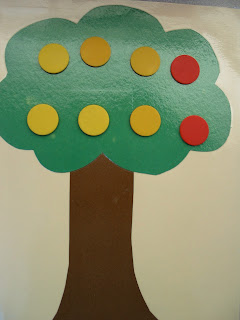## Wednesday, January 16, 2013

### Math Trains and Number Stories

We have been working on creating math trains and number stories.  We have also worked on being able to show multiple ways to show an answer.  It is important for them to be able to explain how they reached the answer!Different ways to show a 6 trian.
Now we are making the math equations to go with the math trains:
3 + 3 = 6
4 + 2 =6
5 + 1 = 6
6 + 0 = 6  etc.Subtraction Number stories...
We have eight apples and two fall off.  How many do we have left?
8 - 2 = 6
We have 6 apples left.Addition Number Stories...
We have 6 yellow apples and 2 red apples.  How many apples do we have all together?
6 + 2 = 8
We have 8 apples all together.

Here is how we practice in Nature...6 + 2 = 84 + 4 = 8Check out our Mascot's Yennadon Shirt!!!